## Definition Of Triangle Proportionality Theorem

Triangle Proportionality Theorem states that a line drawn parallel to any of the sides of a triangle divides the other two sides proportionally.

### Example of Triangle Proportionality Theorem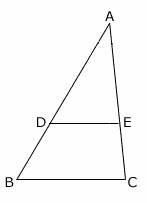In the given triangle ABC, BC is the base of the triangle.
DE is drawn parallel to BC and it intersects the other two sides AB and AC at D and E respectively.
Here,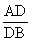=.
This is called triangle proportionality theorem.

### Solved Example on Triangle Proportionality Theorem

#### Ques: QP || BC. If AQ = 3 cm, PC = 4 cm, QB = 11 cm, and BC = 12 cm, then find the length of AP rounded to the nearest decimal.##### Choices:

A. 2.59 cm
B. 1.09 cm
C. 2.09 cm
D. 1.59 cm
Step 2: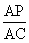=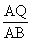[Proportionality Theorem.]
Step 3: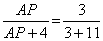[Substitute the values.]